# Understanding I/Q Signals and Quadrature Modulation

## Chapter 5 - Radio Frequency Demodulation

Learn about “I/Q” signals, how they are used, and why they are advantageous in RF systems.

This chapter would not be complete without a page on quadrature demodulation. However, before we explore quadrature demodulation, we need to at least briefly discuss quadrature modulation. And before we discuss quadrature modulation, we need to understand I/Q signals.

The term “I/Q” is an abbreviation for “in-phase” and “quadrature.” Unfortunately, we already have a terminology problem. First of all, “in-phase” and “quadrature” have no meaning on their own; phase is relative, and something can only be “in phase” or “out of phase” with reference to another signal or an established reference point. Furthermore, we now have the word “quadrature” applied to both a signal and the modulation/demodulation techniques associated with that signal.

In any event, “in-phase” and “quadrature” refer to two sinusoids that have the same frequency and are 90° out of phase. By convention, the I signal is a cosine waveform, and the Q signal is a sine waveform. As you know, a sine wave (without any additional phase) is shifted by 90° relative to a cosine wave. Another way to express this is that the sine and cosine waves are in quadrature.

The first thing to understand about I/Q signals is that they are always amplitude-modulated, not frequency- or phase-modulated. However, I/Q amplitude modulation is different from the AM technique discussed in Chapter 4: in an I/Q modulator, the signals that modulate the I/Q sinusoids are not shifted such that they are always positive. In other words, I/Q modulation involves multiplying I/Q waveforms by modulating signals that can have negative voltage values, and consequently the “amplitude” modulation can result in a 180° phase shift. Later in this page we’ll explore this issue in more detail.

What is so advantageous about amplitude-modulating two sinusoids that are 90° out of phase? Why are I/Q modulation and demodulation so widespread? Read on.

### Summing I and Q

I and Q signals on their own are not very interesting. The interesting thing happens when I and Q waveforms are added. It turns out that any form of modulation can be performed simply by varying the amplitude—only the amplitude—of I and Q signals, and then adding them together.

If you take I and Q signals of equal amplitude and add them, the result is a sinusoid with a phase that is exactly between the phase of the I signal and the phase of the Q signal.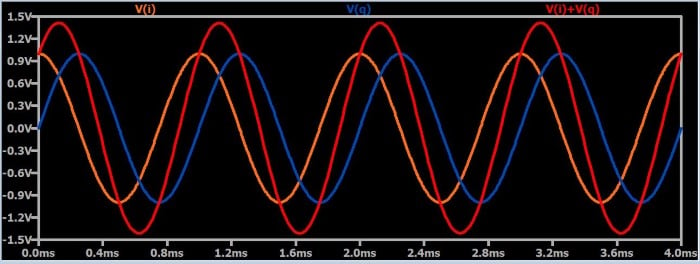In other words, if you consider the I waveform to have a phase of 0° and the Q waveform to have a phase of 90°, the summation signal will have a phase of 45°. If you want to use these I and Q signals to create an amplitude-modulated waveform, you simply amplitude modulate the individual I and Q signals. Obviously a signal will increase or decrease in amplitude if it is created by adding together two signals that are both increasing or both decreasing in amplitude. However, you must ensure that the amplitude modulation applied to the I signal is identical to the amplitude modulation applied to the Q signal, because if they are not identical, you will have phase shift. And that brings us to the next property of I/Q signaling.

### From Amplitude to Phase

Phase modulation, in the form of phase shift keying, is an important technique in modern RF systems, and phase modulation can be conveniently achieved by varying the amplitude of I/Q signals. Consider the following plots: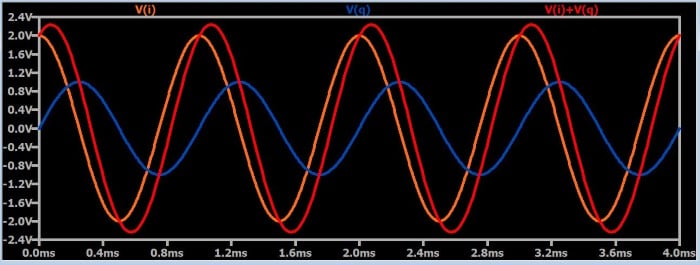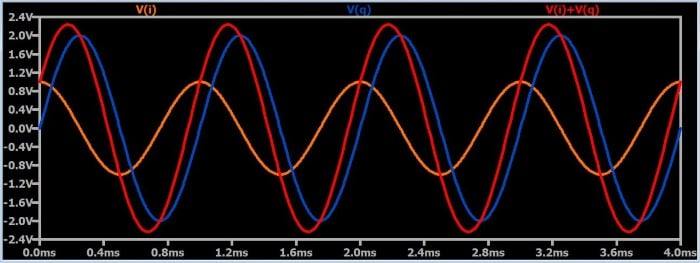As you can see, increasing the amplitude of one of the waveforms relative to the other causes the summation signal to shift toward the higher-amplitude waveform. This makes intuitive sense: if you eliminated the Q waveform, for example, the summation would shift all the way over to the phase of the I waveform, because (obviously) adding the I waveform to zero will result in a summation signal that is identical to the I waveform.

It would seem from the above discussion that I/Q signaling can only be used to shift a signal 90° (i.e., 45° in each direction): if the Q amplitude is reduced to zero, the summation goes all the way to the I phase; if the I amplitude is reduced to zero, the summation goes all the way to the Q phase. How, then, could we use I/Q signals to create (for example) quadrature phase shift keying (QPSK), which uses phase values covering a range of 270°? We’ll discuss this in the next section.

The term “quadrature modulation” refers to modulation that is based on the summation of two signals that are in quadrature. In other words, it is I/Q-signal-based modulation. We’ll use QPSK as an example of how quadrature modulation works, and in the process we’ll see how amplitude modulation of I/Q signals can produce phase shifts beyond 90°.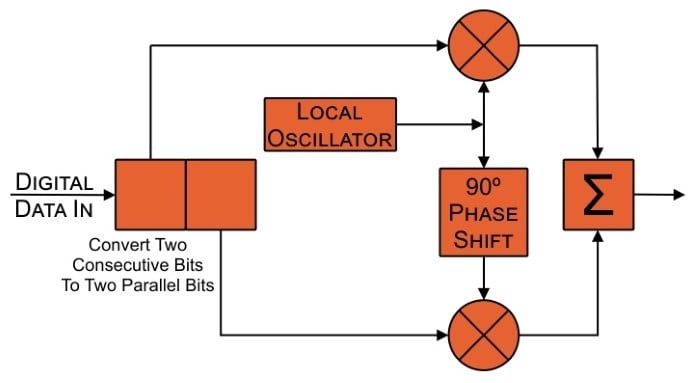This is a basic block diagram for a QPSK modulator. First, the digital data stream is processed so that two consecutive bits become two parallel bits. Both of these bits will be transmitted simultaneously; in other words, as mentioned in this page, QPSK allows one symbol to transfer two bits. The local oscillator generates the carrier sinusoid. The local oscillator signal itself becomes the I carrier, and a 90° phase shift is applied to create the Q carrier. The I and Q carriers are multiplied by the I and Q data streams, and the two signals resulting from these multiplications are summed to produce the QPSK-modulated waveform.

The I and Q data streams are amplitude-modulating the I and Q carriers, and as explained above, these individual amplitude modulations can be used to produce phase modulation in the final signal. If the I and Q data streams were typical digital signals extending from ground to some positive voltage, we would be applying on-off keying to the I and Q carriers, and our phase shift would be restricted to 45° in either direction. However, if the I and Q data streams are bipolar signals—i.e., if they swing between a negative voltage and a positive voltage—our “amplitude modulation” is actually inverting the carrier whenever the input data is logic low (because the negative input voltage multiplied by the carrier results in inversion). This means that we will have four I/Q states:

• I normal and Q normal
• I normal and Q inverted
• I inverted and Q normal
• I inverted and Q inverted

What will summation produce in each of these cases? (Note that in the following plots the frequency of the waveforms is chosen such that the number of seconds on the x-axis is the same as the phase shift in degrees.)

I Normal and Q Normal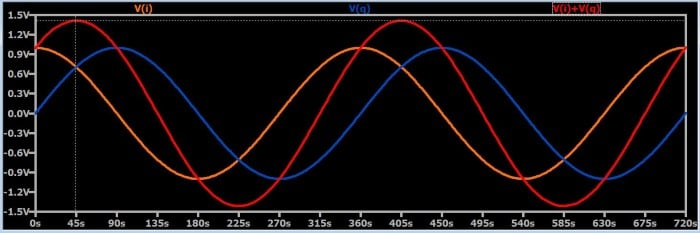I Normal and Q Inverted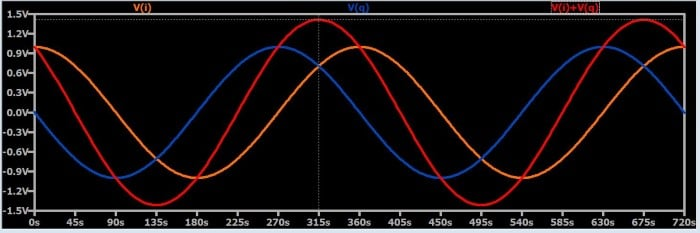I Inverted and Q Normal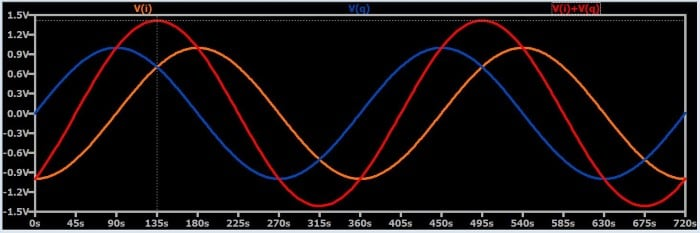I Inverted and Q Inverted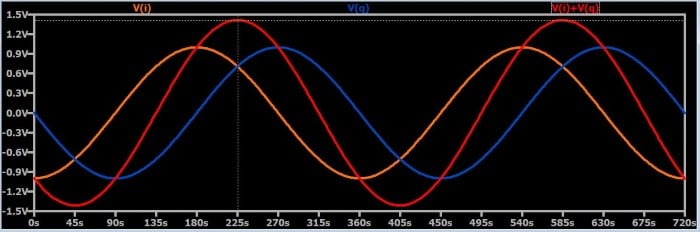As you can see, summation in these four cases produces exactly what we want to have in a QPSK signal: phase shifts of 45°, 135°, 225°, and 315°.

### Summary

• I/Q signaling refers to the use of two sinusoids that have the same frequency and a relative phase shift of 90°.
• Amplitude, phase, and frequency modulation can be performed by summing amplitude-modulated I/Q signals.
• Quadrature modulation refers to modulation that involves I/Q signals.
• Quadrature phase shift keying can be accomplished by adding I and Q carriers that have been individually multiplied, in accordance with the incoming digital data, by +1 or –1.
• Share
Published under the terms and conditions of the Design Science License• E
Eashu January 24, 2020

The summing of I and Q signals result in a signal which is both amplitude and phase modulated.It is actually QAM technique!But you have mentioned “QPSK”.QPSK signals are only phase modulated.Kindly correct me if I am wrong.

Like.
•RK37 September 11, 2020
The plots at the end of the article show how summation of inverted and non-inverted I and Q signals can produce phase shift without amplitude variations.
Like.
•Vitor Augusto April 02, 2020

An example of generating an IQ signal (real) in MATLAB is as follows. In this example, a chirp signal is generated, its phase is put in IQ, then phase is sent and received, then the chirp signal is reconstructed.
>> t=0:1e-8:2e-6;
F0=0;
F1=2e6;
Y = chirp(t,F0,t(end),F1);
Z=exp(j*asin(Y));
IQ=real(Z.*exp(j*2*pi*10e6*t));
a=real(hilbert(IQ).*exp(-j*2*pi*10e6*t));
b=imag(hilbert(IQ).*exp(-j*2*pi*10e6*t));
plot(sin(angle(a+b*j)))
hold on
plot(Y,”—”)

Like.
•peregrin5 September 10, 2020

I did a little bit of editing to some geogebra simulations online to create this IQ modulation phasor to wave simulation which might be fun to play with. I am just learning this concept myself so maybe there are errors. https://www.geogebra.org/m/nwweypcr

Like.